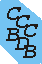Computational Chemistry Comparison and Benchmark DataBase Release 22 (May 2022) Standard Reference Database 101 National Institute of Standards and Technology Home All data for one species Geometry Experimental Calculated Comparisons Bad Calculations Tutorials and Explanations Vibrations Experimental Calculated Scale factors Reactions Entropies Ions List Ions Energy Electron Affinity Proton Affinity Ionization changes point group Experimental One molecule all properties One property a few molecules Geometry Vibrations Energy Electrostatics Reference Data Calculated Energy Optimized Reaction Internal Rotation Orbital Nuclear repulsion energy Correlation Ion Excited State Basis Set Extrapolation Geometry Vibrations Frequencies Zero point energy (ZPE) Scale Factors Bad Calculations Electrostatics Charges Dipole Quadrupole Polarizability Spin Entropy and Heat Capacity Reaction Lookup by property Comparisons Geometry Vibrations Energy Entropy Electrostatics Ion Resources Info on Results Calculations Done Basis functions used I/O files Glossary Conversion Forms Links NIST Links External links Thermochemistry Tutorials Vibrations Entropy Energy Electrostatics Geometry Cost Bad Calculations FAQ Help Units Choose Units Explanations Credits Just show me Summary Using List Recent molecules Molecules Geometry Vibrations Energy Similar molecules Ions, Dipoles, etc. Index of CCCBDB Feedback You are here: Resources > Bad Calculations > Oddities > NaCN Is not linear

# NaCN is not linear

Assuming similarity to H-C≡N (hydrogen cyanide), which is linear, it is reasonable to assume gas-phase Na-C≡N would be linear too. An isomer of H-C≡N exists, C≡N-H (hydrogen isocyanide, also linear) which is approximately 62 kJ mol-1 higher in energy. For Na-C≡N there is a very marginally stable structure for the C≡N-Na geometry. It is close to the same energy (within 10 kJ mol-1) of the Na-C≡N geometry. However the lowest energy (most stable) geometry is triangular with the Na almost perpendicular to the C≡N bond.